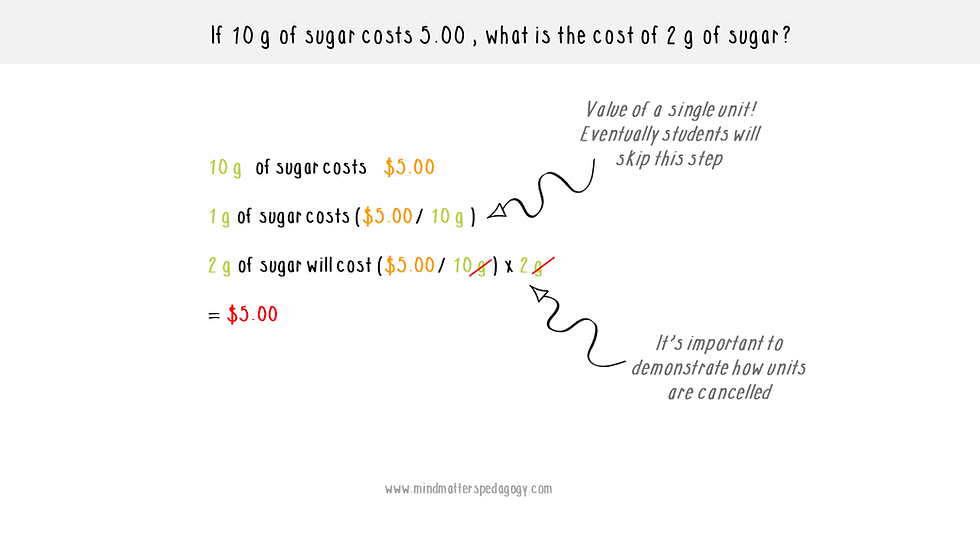top of page
• Androy

# How to Teach Mole Calculations to Students who hate FormulasIn my previous post, I spoke about the use of formula triangles to teach mole calculations to students who hate math. These are useful for students who are not comfortable manipulating and rearranging equations.

However, there are some students who do not like dealing with formulas or symbols and instead prefer clearly written statements outlining the steps needed to solve to problem.

This is where the unitary method can be useful.

## What is the unitary method?

The unitary method is a method used in mathematics in which you find the value of a single unit and then the value of a required number of units. It is a technique used as early as primary school, therefore, most students would be familiar with it by the time they are ready to tackle more complex chemistry problems.

I should mention that this was my preferred method as a student and is usually how I introduce mole calculations to my students. Once I have covered this, then I introduce the other techniques such as mole triangles and allow students to select their preferred method.

## How to Teach the Unitary Method

### 1. Start simple with relatable problems

When I’m introducing this method, I like to show students that they already know how to manipulate the numbers by starting with a very basic problem.

If 10 g of sugar costs \$5.00, what is the cost of 2 g of sugar?

At this point, my students will start to moan and groan asking why they are being given “baby problems” in chemistry class.

However, the goal of these types of questions is to help students gain confidence and to establish a pattern of thinking.### Things to Keep in Mind

1. It is a good practice to have students write the known on the left-hand side of the page, and the unknown on the right-hand side. This should be kept consistent whenever they are doing calculations of this nature to avoid confusion.

2. You should spend time showing students how to cancel off units. This is a good way for them to self-evaluate. I tell my students, "if you don't end up with the unit that you want, then you did something wrong."

Once students get the hang of these types of problems I switch it up to include some moles.

### 2. Switch things up with simple problems involving moles and mass

Students will get the gist of these problems very very quickly. At this point, (after I have explained moles and molar mass and all the prerequisite content) I throw in some mass to moles conversions.

Calculate the number of moles of carbon (atoms) in 24 g of Carbon.

Allow students to figure this out on their own. If they were paying attention during the "baby problems" they should quickly realize that these are done in the exact same way as before.### Things to Keep in Mind

1. Ensure that students work on these problems in the same format as before. Initially, this would include the step where they calculate the value of a single unit, do not allow them to skip this step until they are more comfortable with these calculations.

2. Remind students that we can always calculate the mass of 1 mole of any substance as long as the chemical formula is known.

3. Have students work out the units every single time

### 3. Introduce more complex Questions

Once students are comfortable doing calculations involving simple conversions such as:you can start introducing more complex problems.

The unitary method works beautifully for calculations involving concentrations of solutions.Notice how these calculations follow the exact same format before with problem statements clearly written out.

Remind students that a concentration of 0.100 mol/dm3 means that there is 0.100 mol in 1dm3 of solution or 1000cm3 of solution.

## Why I prefer the unitary method over all the other techniques for mole calculations

1. The required math is basic. Once students understand how to find the value of one unit, everything else is pretty straightforward.

2. When using this method, students do not have to retrieve any equations or triangles from memory. By using this technique, they can logically work their way through any problem.

3. Because they are using problem statements students develop a better understanding of the question and what is required, and they can easily keep track of their calculations. With dimensional analysis and the mole formulas, some students become confused with all the numbers and symbols on the page that may appear disconnected from the question.

## Expose students to different techniques for solving these problems

It is important that students are exposed to a number of different techniques for solving mole problems. Students' learning styles should be taken into account when teaching and also when selecting resources to aid learning.

One size does not fit all, and students become a lot more confident when they are allowed to use the techniques that they feel comfortable with for solving problems in chemistry.

Over time, you will notice students begin to use an amalgamation of the different techniques to solve more complex problems, such as using the formula for simple mole conversions and the unitary method for solving problems with solutions.

If your students are comfortable using dimensional analysis, the equations, or the unitary method alone, then that’s fine, every classroom is different. However, if you notice some of your students really struggling with the one method you chose, maybe it's time to switch things up.

Do you use the unitary method in your classroom? If not what problem-solving technique do you use?bottom of page